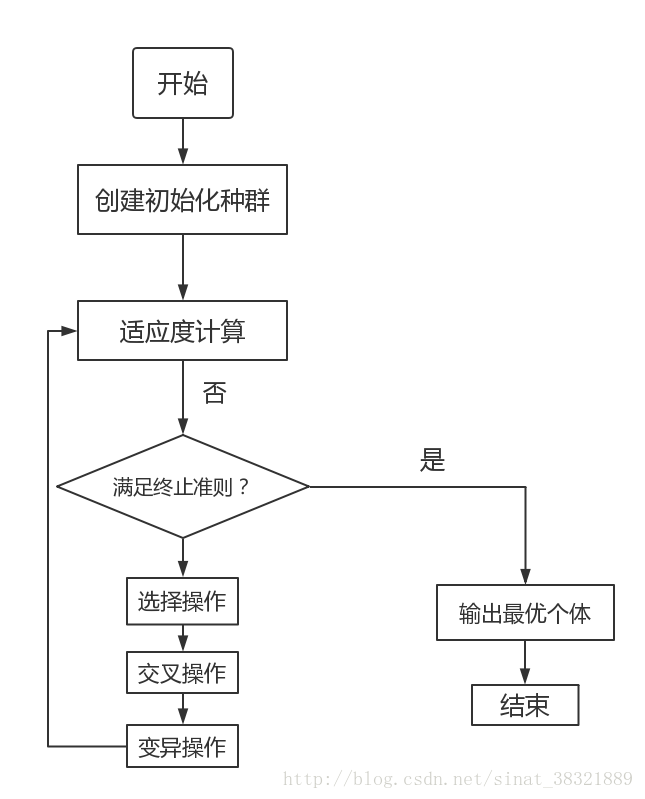# 遗传算法

## 基本概念

1. 染色体：待解决的数学问题的一个可行解成为染色体。
2. 基因：一个可行解一般由多个元素构成，那么这每一个元素就被称为染色体上的一个基因。
3. 适应度函数：执行优胜劣汰的函数。将适应度高的染色体留下，将适应度低的染色体淘汰掉。从而经过若干次迭代后染色体的质量将越来越优良。
4. 交叉：两个染色体生成一个新的染色体，新染色体上的基因由轮盘赌算法完成。在每完成一次进化后，都要计算每一条染色体的适应度，然后采用公式计算每一条染色体的适应度概率。那么在进行交叉过程时，就需要根据这个概率来选择父母染色体。适应度比较大的染色体被选中的概率就越高。这也就是为什么遗传算法能保留优良基因的原因。（染色体i被选择的概率 = 染色体i的适应度 / 所有染色体的适应度之和）
5. 变异：交叉能保证每次进化留下优良的基因，但它仅仅是对原有的结果集进行选择，基因还是那么几个，只不过交换了他们的组合顺序。这只能保证经过N次进化后，计算结果更接近于局部最优解，而永远没办法达到全局最优解，为了解决这一个问题，我们需要引入变异。变异很好理解。当我们通过交叉生成了一条新的染色体后，需要在新染色体上随机选择若干个基因，然后随机修改基因的值，从而给现有的染色体引入了新的基因，突破了当前搜索的限制，更有利于算法寻找到全局最优解。
6. 选择：淘汰fw个体，选出优秀个体传给下一代。
7. 表现型：输入的值。
8. 基因型：将输入值编码后的值。故表现型通过编码变成基因型，基因型通过解码变成表现型。
9. 编码方式：分为二进制编码，实数编码和符号编码。

## 算法

### 流程

1. 在算法初始阶段，它会随机生成一组可行解，也就是第一代染色体。
2. 然后采用适应度函数分别计算每一条染色体的适应程度，并根据适应程度计算每一条染色体在下一次进化中被选中的概率。
3. 通过“交叉”，生成N-M条染色体；
4. 再对交叉后生成的N-M条染色体进行“变异”操作；
5. 然后使用“复制”的方式生成M条染色体；## 代码

### 步骤

1. 初始化种群
2. 计算目标函数值和适应度值：目标函数的结果可以是负数，但是适应度值不能为负数。因为后面会用轮盘赌法算概率，如果适应度值为负数的话，概率也为负数。母猪会上树概率都不可能为负数。所以，要进行处理，当目标函数为负数时候适应度值要为0。
3. 选择，交叉，变异
4. 求最优个体，并记录
5. 继续下一次迭代，直到迭代结束
6. 根据每一次迭代的最优个体，再找出其中的最优个体，即为最优解

### 主程序

clear
clc
popsize=20;                         %群体大小
chromlength=10;                  %字符串长度(个体长度)
pc=0.6;                     %交叉概率，只有在随机数小于pc时，才会产生交叉
pm=0.001;                           %变异概率
times = 2000                % 遗传次数

pop=initpop(popsize,chromlength);               %随机产生初始群体
for i=1:times
[objvalue]=calobjvalue(pop);                  %计算目标函数
fitvalue=calfitvalue(objvalue);    %计算群体中每个个体的适应度

[newpop]=selection(pop,fitvalue);             % 选择
[newpop1]=crossover(newpop,pc);               % 交叉
[newpop2]=mutation(newpop1,pm);               % 变异

[objvalue]=calobjvalue(newpop2);     %计算目标函数
fitvalue=calfitvalue(objvalue);   %计算群体中每个个体的适应度

[bestindividual,bestfit]=best(newpop2,fitvalue); %求出群体中适应值最大的个体及其适应值
y(i)=bestfit;   %返回的 y 是自适应度值，而非函数值
x(i)=decodebinary(bestindividual)*10/1023; %将自变量解码成十进制
pop=newpop2;
end
fplot('x+10*sin(5*x)+7*cos(4*x)',[0 10])
hold on
plot(x,y,'r*')
hold on

[z ,index]=max(y);             % 计算所有最优良个体中的最优良个体
x5=x(index)                    % 计算最大值对应的x值
ymax=z


### 初始化 initpop.m

function pop=initpop(popsize,chromlength)
pop=round(rand(popsize,chromlength));


### 计算目标函数值 calobjvalue.m

function [objvalue]=calobjvalue(pop)
temp1=decodebinary(pop);   % 将pop每行转化成十进制数
x=temp1*10/1023;      % 得到十进制数在0~1023之间，通过变换得到x属于0~10的值
objvalue=x+10*sin(5*x)+7*cos(4*x);   % 通过x的值计算目标函数值


### 二进制转换为十进制 decodebinary.m

sum函数第二个参数不写默认是1，表示对每一列求和得到行向量。如果第二个参数输入2，则表示对每一行求和得到列向量。

function pop2=decodebinary(pop)
[px,py]=size(pop);                   %求pop行和列数
for i=1:py
pop1(:,i)=2.^(py-i).*pop(:,i);
end
pop2=sum(pop1,2);        %求pop1的每行之和


### 适应度函数 calfitvalue.m

function fitvalue=calfitvalue(objvalue)

[px,py]=size(objvalue);                   %目标值有正有负
for i=1:px
if objvalue(i)>0
temp=objvalue(i);
else
temp=0.0;
end
fitvalue(i)=temp;
end
fitvalue=fitvalue';


### 选择 selection.m

function [newpop]=selection(pop,fitvalue)
totalfit=sum(fitvalue);                   %求适应值之和
fitvalue=fitvalue/totalfit;       %单个个体被选择的概率，轮盘赌算法的公式
fitvalue=cumsum(fitvalue);      % 累加是为了将分布律转换为分布函数然后可以比较
[px,py]=size(pop);                       %20*10
ms=sort(rand(px,1));                   % 将随机数从小到大排列
fitin=1;
newin=1;
while newin<=px       % 直到20个随机数被走完才跳出循环
if(ms(newin))<fitvalue(fitin)   % 概率小于分布函数，优良个体被选择
newpop(newin,:)=pop(fitin,:);
newin=newin+1;
else             % 概率大于分布函数，个体被淘汰，继续选择下一个个体
fitin=fitin+1;
end
end


### 交叉 crossover.m

function [newpop]=crossover(pop,pc)    % pc=0.6 pc是交叉概率
[px,py]=size(pop);    % px是种群数量，py是编码长度
newpop=ones(size(pop));   % 生成一群全为1的种群
for i=1:2:px-1        % 步长为2，是将相邻的两个个体进行交叉
if(rand<pc)     % 如果随机数小于交叉概率，则发生了交叉
cpoint=round(rand*py);    % cpoint: 0-10的随机数
newpop(i,:)=[pop(i,1:cpoint),pop(i+1,cpoint+1:py)]; % 交叉操作
newpop(i+1,:)=[pop(i+1,1:cpoint),pop(i,cpoint+1:py)];  % 交叉操作
else    % 没有发生交叉newpop保持原有pop的个体
newpop(i,:)=pop(i,:);
newpop(i+1,:)=pop(i+1,:);
end
end


### 变异 mutation.m

function [newpop]=mutation(pop,pm)    % pop是种群，pm是变异概率
[px,py]=size(pop);
newpop=ones(size(pop));
for i=1:px
if(rand<pm)      % 随机数小于变异概率，发生了变异
mpoint=round(rand*py);     % 产生的变异点在1-10之间
if mpoint<=0  % 可以写成 mpoint == 0 ，因为这个值不可能小于0，但是可以等于0
mpoint=1;                % mpoint表示变异位置
end
newpop(i,:)=pop(i,:);
if any(newpop(i,mpoint))==0% 这个any意义不大，因为传入的是一个值，不是矩阵
newpop(i,mpoint)=1;     % 把0变异成1
else
newpop(i,mpoint)=0;    % 或者把1变异成0
end
else                          % 不发生变异，原来的个体还是原来的个体
newpop(i,:)=pop(i,:);
end
end


### 求最大适应度个体 best.m

function [bestindividual,bestfit]=best(pop,fitvalue)
[px,py]=size(pop);
bestindividual=pop(1,:);
bestfit=fitvalue(1);
for i=2:px
if fitvalue(i)>bestfit
bestindividual=pop(i,:);
bestfit=fitvalue(i);
end
end


## 自己的一些感悟

1. 关于交叉：0-1编码的交叉是截取染色体部分进行交叉，实数编码的交叉是所有染色体进行随机权值相乘交叉。

2. 关于选择：如果适应度是越小越好可以将适应度取倒数（或者用一个常数除以这个数，比如10）然后再用轮盘赌算法。

3. 关于变异：0-1编码的变异是将1变成0，将0变成1。实数编码就是将该数加上或减去该数与上界或下界的差或和（这个随机判定）再乘以一个比较小的数，记得实数编码要检验是否在规定边界范围内。0-1编码不用检测。关于变异有一套代码：

fg = (rand*(1-num/maxgen))^2;  % num：当前迭代次数。maxgen：最大迭代次数
if pick > 0.5
% chrom：种群。i：第i个个体索引。pos：变异的位置
chrom(i, pos) = chrom(i, pos)+(chrom(i, pos)-bound(pos, 2))*fg;
else
chrom(i, pos) = chrom(i, pos)+(bound(pos, 1)-chrom(i, pos))*fg;
end


## 参考文章

1. 十分钟搞懂遗传算法：https://zhuanlan.zhihu.com/p/33042667

2. 遗传算法介绍并附上matlab代码:https://www.cnblogs.com/LoganChen/p/7509702.html

3. matlab遗传工具包：https://zhuanlan.zhihu.com/p/158600868

4. 一个非常好的理解遗传算法的例子 强烈推荐入门：https://blog.csdn.net/u012422446/article/details/68061932

5. 【算法】超详细的遗传算法(Genetic Algorithm)解析：https://www.jianshu.com/p/ae5157c26af9

6. 遗传算法(Genetic Algorithm)原理详解和matlab代码解析实现及对应gaot工具箱实现代码https://blog.csdn.net/qq_35608277/article/details/83785678

posted @ 2021-09-29 09:33  nerd`  阅读(119)  评论(0编辑  收藏  举报